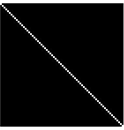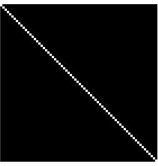## Search This Blog

#Day35-Left to right diagonal in an image

Print a diagonal from left to right in white colour, with a black background as shown below. Take user input for the number of rows.Note: This code can be done using the in-built command. But for the challenge, I am trying to avoid those

Matlab Code:

function image_lefttorightdiagonal(x)

for i=1:1:x

for j=1:1:i

a(i,j)=0;

end

a(i,j)=225;

fprintf('\n');

end

imshow(a);

Sample Input and Output

image_lefttorightdiagonal(70)Live Support: https://www.t.me/matlabcastor Share with us: https://www.quora.com/q/matlabirawen Free Codes: https://www.youtube.com/castorclasses Free Support: https://www.castorclasses2014@gmail.com Like us: https://www.facebook.com/matlabirawen/ Join us: https://www.facebook.com/groups/MATLA... MATLAB Book for the beginner: https://amzn.to/3fTfmTa Free program: https://www.matlabcoding.com

/>

MATLAB